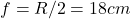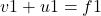## A small candle, 2.5 cm in size is placed at 27 cm in front of a concave mirror of radius of curvature 36 cm. At what distance from the mirro

Question

A small candle, 2.5 cm in size is placed at 27 cm in front of a concave mirror of radius of curvature 36 cm. At what distance from the mirror should a screen be placed to obtain a sharp image? Describe the nature and size of the image. If the candle is moved closer to the mirror, how would the screen have to be moved?

in progress 0
6 months 2021-08-08T14:18:59+00:00 1 Answers 2 views 0

## Answers ( )

1. If the candle is moved closer to the mirror, then the screen will have to be moved away from the mirror in order to obtain the image.

Explanation:

Object distance, u=−27cm

Radius of curvature of the concave mirror, R=−36cm

focal length of mirror f=R/2=−18cmThe image distance can be obtained using the mirror formula,From above equation, v=  −54cm.

Therefore, the screen should be placed 54 cm away from the mirror to obtain a sharp image. The magnification of the image is given as,∴h′= −5cm

The height of the candle’s image is 5 cm. The negative sign indicates that the image is inverted and virtual. If the candle is moved closer to the mirror, then the screen will have to be moved away from the mirror in order to obtain the image.